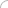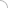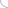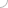# Races and games - aptitude test

Home > Aptitude test > Races and games

##### 1) If in a race of 80m, A covers the distance in 20 seconds and B in 25 seconds, then A beats B by:

a) 20m
b) 16m
c) 11m
d) 10m

SOLUTION :

The difference in the timing of A and B is 5 seconds. Hence, A beats B by 5 seconds.
The distance covered by B in 5 seconds = (80 * 5) / 25 = 16m
Hence, A beats B by 16m.

##### 2) If X can run 48m and Y 42m, then in a race of 1km, X beats Y by:

a) 140m
b) 125m
c) 100m
d) 110m

SOLUTION :

When X runs 48m, Y runs 42m.
Hence, when X runs 1000m, Y runs (1000 * 42) / 48 = 875m
X beats Y by 125m.

##### 3) In a race of 800m, if the ratio of the speeds of two participants P and R is 3:4, and P has a start of 120m, then R beats P by:

a) 100m
b) 80m
c) 90m
d) 110m

SOLUTION :

As P has a start of 120m, P has to cover 680m while R has to cover 800m.
Now, when P covers 3m, R covers 4m.
Hence, when R covers 800m, P covers (800 * 3) / 4 = 600m
Therefore, R beats P by 80m.

##### 4) If in a race of 120m X can beat Y by 20m and Z by 35m, then Y can beat Z by:

a) 12m
b) 10m
c) 15m
d) 18m

SOLUTION :

In a race of 120m, as X beats Y by 20m, when X runs 120m, Y runs 100m.
Similarly, as X beats Z by 35m, when X covers 120m, Z covers 85m.
Hence, when Y runs 100m, Z runs 85m.
When Y runs 120m, Z runs (120 * 85) / 100 = 102m
Hence, Y beats Z by 18m.

##### 5) If in a game of 80, P can give 16 points to Q and R can give 20 points to P, then in a game of 150, how many points can R give to Q?

a) 48
b) 60
c) 54
d) 90

SOLUTION :

When P scores 80, Q scores 64.
When R scores 80, P scores 60
Hence, when R scores 150, Q scores (60 * 64 * 150) / (80 * 80) = 90
Therefore, in a game of 150, R can give 60 points to Q.

 Discussion Board Unable to view formalae section in apptitude Some times im able to see the formulas some times I''m only able to see practice questions ,please make how to get the formulas page in apptitiude roy 12-19-2021 11:05 AMAptitude questions